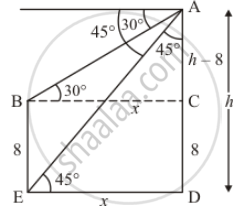Share

# The Angles of Depression of the Top and Bottom of 8 M Tall Building from the Top of a Multistoried Building Are 30° and 45° Respectively. Find the Height of the Multistoried Building and the Distance Between the Two Buildings. - CBSE Class 10 - Mathematics

#### Question

The angles of depression of the top and bottom of 8 m tall building from the top of a multistoried building are 30° and 45° respectively. Find the height of the multistoried building and the distance between the two buildings.

#### Solution

Let AD be the multistoried building of height hm. And the angle of depression of the top and bottom are 30° and 45°. We assume that BE = 8, CD = 8 and BC = xED = x and AC = h − 8. Here we have to find height and distance of the building.

We use trigonometric ratio.In ΔAED,

=> tan E = (AD)/(DE)

=> tan 45^@= (AD)/(DE)

=> 1 = h/x

=> x = h

Again in Δ ABC

=> tan B = (AC)/(BC)

=> tan 30^@ = (h - 8)/x

=> 1/sqrt3 = (h - 8)/x

=> hsqrt3 - 8sqrt3 = x

> hsqrt3 - 8sqrt3 =  h

=> h(sqrt3 - 1) = 8sqrt3

=> h = (8sqrt3)/(sqrt3 - 1) xx (sqrt3 + 1)/(sqrt3 + 1)

=> h = (24 + 8sqrt3)/2

=> h = (4(3 + sqrt3))

And

=> x = 4(3 + sqrt3)

Hence the required height is  4(3 + sqrt3) meter and distance is 4(3 + sqrt3) meter

Is there an error in this question or solution?

#### Video TutorialsVIEW ALL 

Solution The Angles of Depression of the Top and Bottom of 8 M Tall Building from the Top of a Multistoried Building Are 30° and 45° Respectively. Find the Height of the Multistoried Building and the Distance Between the Two Buildings. Concept: Heights and Distances.
S